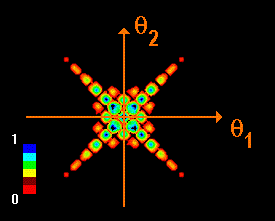﻿ Bosons
Quantum Mechanics
Identical particles: Bosons

Two non-interacting identical bosons can occupy the same point in space, or they can have the same value of momentum. The picture on the right shows a probability distribution of two identical bosons.The animations show the time development of the probability distribution of the two non-interacting bosons. To illustrate the differences between the fermions and the bosons, the latter are also assumed to carry electrical charge. Upper part: Double-slit (in white), no magnetic field. Lower part: Double-slit (in white), confined magnetic field (in red). The magnetic flux through the red area is chosen such that for a single particle the shift in the diffraction pattern due to the Aharonov-Bohm effect is 180°.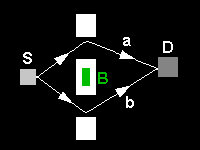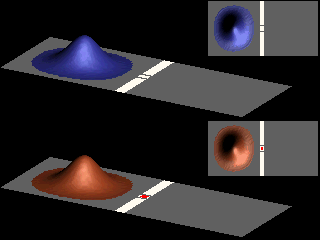The animation shows the interchange of the maxima and minima of the probability distribution, due to the Aharonov-Bohm effect.

The salient features of the intensity measured by detector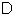are the same as for the case where the source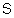emits a single particle.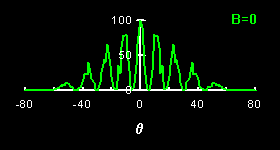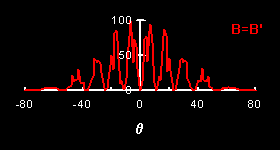Comparing the angular distributions for fermions and bosons shows that, due to the exclusion principle, the interference fringes of the former are less pronounced, as illustrated in the next figure.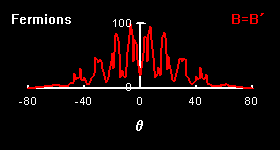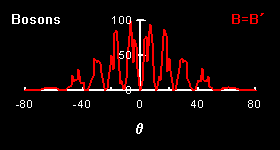The bosonic nature of the wave reveals itself in the cross-correlated signal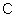of the detectorsand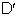.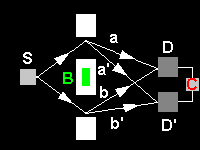Just as for a detector measuring the angular distribution, the positions of the detectors are specified by the angles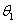and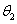.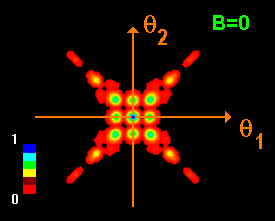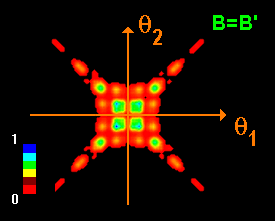The symmetry of the wave function leads to an increase of the probability for finding two bosons close to each other. Indeed, the left picture shows that the probability is the largest for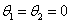, i.e. if the bosons are on top of each other. The correlated two-particle signal also shows the Aharonov-Bohm effect, as can be clearly seen by substracting the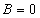and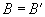signals.